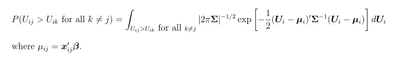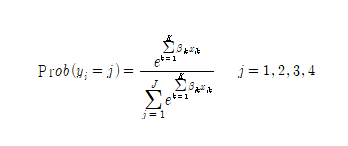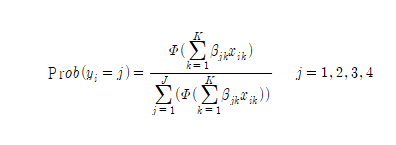## Multinomial Probit Predicted Value

Hi, I am Kyungjae Lee.

I analyzed a multinomial probit model using Proc MDC.

My model is as of mode choice. There are four categories: bus train multi car(ref.).

My code is as following:

proc mdc data=con05 outest=aaa covout; where area1=11 and c_area1=11;
model decision= bus train multi
age_bus gender_bus marry_bus school2_bus school3_bus status2_bus status3_bus own_bus htype2_bus htype3_bus car_own_bus
age_train gender_train marry_train school2_train school3_train status2_train status3_train own_train htype2_train htype3_train car_own_train
age_multi gender_multi marry_multi school2_multi school3_multi status2_multi status3_multi own_multi htype2_multi htype3_multi car_own_multi
/ nchoice=4 type=mprobit maxiter=10000000 unitvariance=(1 2 3 4);
id subject;
output out=abc pred=p xbeta=xbr;
run;

I could find the predicted value by inputting this code: output out=abc pred=p.

However, I want to know how to calculate the predicted value.

I tried to find out an equation to calculate it, but I could not find it.

I will really appreciate if you let me know the exact equation.

Thank you.

1 ACCEPTED SOLUTION

Accepted Solutions

## Re: Multinomial Probit Predicted Value

Yes, there is a simple formula for the multinomial logit model, but there is no such formula for multinomial probit. The predicted value is the choice probability, P(U_ij > U_ik for all k not equal to j), which is defined by an integral. In the multinomial logit model, this integral can easily be solved in closed form, which is why there is a simple formula. In the multinomial probit model, the integral is given by:This integral is analytically intractable - there is no simple formula. So instead we have to approximate it using a numerical integration technique. The GHK simulator is the method MDC uses to approximate the integral. It is quite complex and I do not recommend that you try to do it yourself.

5 REPLIES 5

## Re: Multinomial Probit Predicted Value

In general for the multinomial discrete choice models in MDC, the predicted value is the probability that the alternative is chosen, conditional on the the model, the parameter estimates, and the covariates. For individual i and alternative j this is given by:

pred_ij = P( U_ij > U_ik for all k not equal to j)

where U_ik is the estimated utility for individual i for alternative j. For multinomial probit, these probabilities cannot be computed in closed form. So instead, MDC simulates from the joint distribution of i's utilities, and uses that simulation to approximate the predicted probabilities. Simulation is easy because the vector of utilities are multivariate normal distributed with a known mean vector (given by the xbetas), and a known covariance matrix (given by the estimate of Sigma). The particular simulation technique MDC uses is known as the GHK simulator. The MDC documentation has some references discussing the GHK simulator that you can read for more information:

• Geweke, J. (1989). “Bayesian Inference in Econometric Models Using Monte Carlo Integration.” Econometrica 57:1317–1339.

• Hajivassiliou, V. A. (1993). “Simulation Estimation Methods for Limited Dependent Variable Models.” In Econometrics, edited by G. S. Maddala, C. R. Rao, and H. D. Vinod. Vol. 11 of Handbook of Statistics, 519–543. New York: Elsevier Science.

• Keane, M. P. (1994). “A Computationally Practical Simulation Estimator for Panel Data.” Econometrica 62:95–116.

• Hajivassiliou, V. A., McFadden, D. L., and Ruud, P. A. (1996). “Simulation of Multivariate Normal Rectangle Probabilities and Their Derivatives: Theoretical and Computational Results.” Journal of Econometrics 72:85–134

## Re: Multinomial Probit Predicted Value

I read the papers you recommended but I could not fully understand. Thus, I ask another question.

We can easily calculate predicted(or expected) value(probability of choosing alternative j) when we use a multinomial logit model.

The equation is as following.Then I wonder whether the following equation is suitable for calculating the predicted value(probability of choosing alternative j) when using a multinomial probit model.I really appreciate for your help.

## Re: Multinomial Probit Predicted Value

Yes, there is a simple formula for the multinomial logit model, but there is no such formula for multinomial probit. The predicted value is the choice probability, P(U_ij > U_ik for all k not equal to j), which is defined by an integral. In the multinomial logit model, this integral can easily be solved in closed form, which is why there is a simple formula. In the multinomial probit model, the integral is given by:This integral is analytically intractable - there is no simple formula. So instead we have to approximate it using a numerical integration technique. The GHK simulator is the method MDC uses to approximate the integral. It is quite complex and I do not recommend that you try to do it yourself.

## Re: Multinomial Probit Predicted Value

Also, the integral is actually slightly more complex than what I show, because the utility of the reference alternative is fixed to zero. But this doesn't change the main conclusion: that the integral is analytically intractable, so we have to numerically integrate it to obtain the predictions.